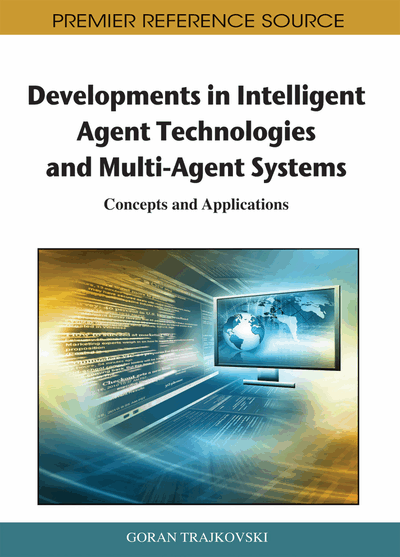# Reward Shaping and Mixed Resolution Function Approximation

Marek Grzes (University of Waterloo, Canada) and Daniel Kudenko (University of York, UK)
DOI: 10.4018/978-1-60960-171-3.ch007
Available
\$37.50
No Current Special Offers

## Abstract

A crucial trade-off is involved in the design process when function approximation is used in reinforcement learning. Ideally the chosen representation should allow representing as closely as possible an approximation of the value function. However, the more expressive the representation the more training data is needed because the space of candidate hypotheses is larger. A less expressive representation has a smaller hypotheses space and a good candidate can be found faster. The core idea of this chapter is the use of a mixed resolution function approximation, that is, the use of a less expressive function approximation to provide useful guidance during learning, and the use of a more expressive function approximation to obtain a final result of high quality. A major question is how to combine the two representations. Two approaches are proposed and evaluated empirically: the use of two resolutions in one function approximation, and a more sophisticated algorithm with the application of reward shaping.
Chapter Preview
Top

## Introduction

In contrast to supervised learning, RL agents are not given instructive feedback on what the best decision in a particular situation is. This leads to the temporal credit assignment problem, that is, the problem of determining which part of the behaviour deserves the reward (Sutton, 1984). To address this issue, the iterative approach to RL applies backpropagation of the value function in the state space. Because this is a delayed, iterative technique, it usually leads to a slow convergence, especially when the state space is huge. In fact, the state space grows exponentially with each variable added to the encoding of the environment when the Markov property needs to be preserved (Sutton & Barto, 1998).

When the state space is huge, the tabular representation of the value function with a separate entry for each state or state-action pair becomes infeasible for two reasons. Firstly, memory requirements become prohibitive. Secondly, there is no knowledge transfer between similar states and a vast number of states need to be updated many times. The concept of value function approximation (FA) has been successfully used in reinforcement learning (Sutton, 1996) to deal with huge or infinite (e.g., due to continuous variables) state spaces. It is a supervised learning approach which aims at approximating the value function across the entire state space. It maps values of state variables to the value function of the corresponding state.

A crucial trade-off is involved in the design process when function approximation is used. Ideally the chosen representation should allow representing as closely as possible an approximation of the value function. However, the more expressive the representation the more training data is needed because the space of candidate hypotheses is larger (Mitchell, 1997). A less expressive representation has a smaller hypotheses space and a good candidate can be found faster. Even though such a solution may not be particularly effective in terms of the asymptotic performance, the fact that it converges faster makes it useful when applied to approximating the value function in RL. Specifically, a less expressive function approximation results in a broader generalisation and more distant states will be treated as similar and the value function in this representation can be propagated faster. The core idea of this chapter is the use of a mixed resolution function approximation, that is, the use of less expressive FA to provide useful guidance during learning and the use of more expressive FA to obtain a final result of high quality. A major question is how to combine the two representations. The most straightforward way is to use two resolutions in one function approximation. A more sophisticated algorithm can be obtained with the application of reward shaping. The shaping reward can be extracted from a less expressive (abstract) layer and used to guide more expressive (ground) learning.

To sum up: in this chapter we propose combining more and less expressive function approximation, and three potential configurations are proposed and evaluated:

• the combination of less and more expressive representations in one approximation of the value function,

• the use of less expressive function approximation to learn the potential function for reward shaping which is used to shape the reward of learning with desired resolution at the ground level,

• the synergy of the previous two, that is, learning the potential function from less expressive approximation and using it to guide learning which combines less and more expressive resolution in one FA at the ground level.

Our analysis of these ideas is based on tile coding (Lin & Kim, 1991) which is commonly used for FA in RL. The proposed extensions to RL are however of general applicability and can be used with different methods of function approximation, especially those which use basis functions with local support (Bishop, 1996).

The rest of this chapter is organised as follows. In the next section, function approximation with tile coding is introduced. Learning with mixed resolution tile coding and the algorithm which learns the potential function for reward shaping are discussed in two subsequent sections. Then, the experimental validation of the proposed extensions to RL is presented, and the last section summarises this chapter.

## Complete Chapter List

Search this Book:
Reset